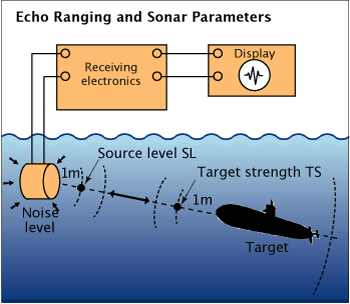# SONAR Equation

The “sonar equation” is a systematic way of estimating the expected signal-to-noise ratios for sonar (SOund Navigation And Ranging) systems. The signal-to-noise ratio determines whether or not a sonar will be able to detect a signal in the presence of background noise in the ocean. It takes into account the source level, sound spreading, sound absorption, reflection losses, ambient noise, and receiver characteristics. The sonar equation is used to estimate the expected signal-to-noise ratios for all types of sonar systems. Slightly different versions of the sonar equation are used for active (echo-ranging) and passive sonar systems.

Active sonar

Active sonar systems, such as fish finders, echosounders, side-scan sonars, and military sonars, transmit a pulse of sound and then listen for echoes. In an active sonar system the source also acts as a receiver.Echo ranging and sonar parameters. Adapted from Urick, 1983.

The sonar equation must account for how loud the sound source is (source level), sound spreading and attenuation as the sound pulse travels from the sonar to the target (transmission loss), the amount of sound reflected back toward the sonar by the target (target strength), sound spreading and attenuation as the reflected pulse travels back to the receiver (transmission loss), the background noise at the receiver (noise level), and the receiver characteristics (array gain). The terms in the sonar equation are in decibels, and they are added together in forming the sonar equation (See Introduction to Decibels).

The sonar transmits a signal with a source level SL, given in underwater dB one meter from the source. The sound becomes weaker as it travels toward the target, due to spreading and absorption. The total reduction in signal intensity is called the transmission loss TL, given in decibels. The sound intensity at the target is then (SL -TL) decibels. Only part of the sound that hits the target, whether it is a school of fish, the sea floor, or a submarine, is reflected back toward the sonar. The intensity of the echo one meter from the target relative to the intensity of the sound hitting the target is called the target strength TS, given in decibels. The echo one meter from the target essentially looks like the signal from a source with a source level of:

Echo intensity (decibels) = (SL – TL) + TS

As the reflected signal travels back to the sonar system, the signal intensity is again reduced by the transmission lossTL. The intensity of the returned signal or echo at the receiver is then:

Returned signal intensity (decibels) = (SL – TL) + TS – TL

which can be simplified to:

Returned signal intensity (decibels) = SL -2TL +TS

If the noise level at the receiver is NL decibels, then the ratio of the signal level to the noise level at the receiver, called the signal-to-noise ratio (SNR), is:

SNR (decibels) = SL -2TL +TS – NL

Large receivers, which are often constructed by assembling an array of smaller receivers, are able to look in a specific direction and reject noise from all other directions. The effective noise level is then reduced by the array gain AG, given in decibels, and the SNR is increased:

SNR (decibels) = SL -2TL +TS – (NL – AG)

An example for a submarine search sonar illustrates the use of the sonar equation to compute the signal-to-noise ratio.

Passive sonar

Passive sonar systems listen to sounds generated by whales, volcanoes, submarines, and other sources of underwater sound, rather than listening to echoes reflected from a target. In this case the sonar equation must account for how loud the sound source is (source level), sound spreading and attenuation as the sound pulse travels from the source to the receiver where it is detected (transmission loss), the background noise at the receiver (noise level), and the receiver characteristics (array gain). (The same sonar equation is used to estimate the expected signal-to-noise ratios for all one-way transmissions, including those used by SOFAR and RAFOS floats and by tomographic systems that measure ocean temperatures and currents.)

The sound source, whether it be a whale, volcano, submarine, or SOFAR float, radiates a signal with a source level SL. The sound intensity is reduced by the transmission loss TL as the sound travels from the source to the receiver. The sound intensity at the receiver is then:

Received signal intensity (decibels) = SL -TL

If the noise level at the receiver is NL decibels, then the ratio of the signal level to the noise level at the receiver, called the signal-to-noise ratio (SNR) is:

SNR (decibels) = SL -TL – NL

Large receivers are able to look in a specific direction and reject noise from all other directions. The effective noise level is then reduced by the array gain AG, given in decibels, and the SNR is increased:

SNR (decibels) = SL -TL – (NL – AG)

The passive sonar equation is much simpler than the active sonar equation because passive sonars do not listen for echoes returning from a target. The term for target strength TS needed for active sonars is therefore irrelevant. The transmission loss TL appears only once, because only one-way transmissions are involved.

An example for the Acoustic Thermometry of Ocean Climate (ATOC) project illustrates the use of the passive sonar equation to compute the signal-to-noise ratio for one-way transmissions.